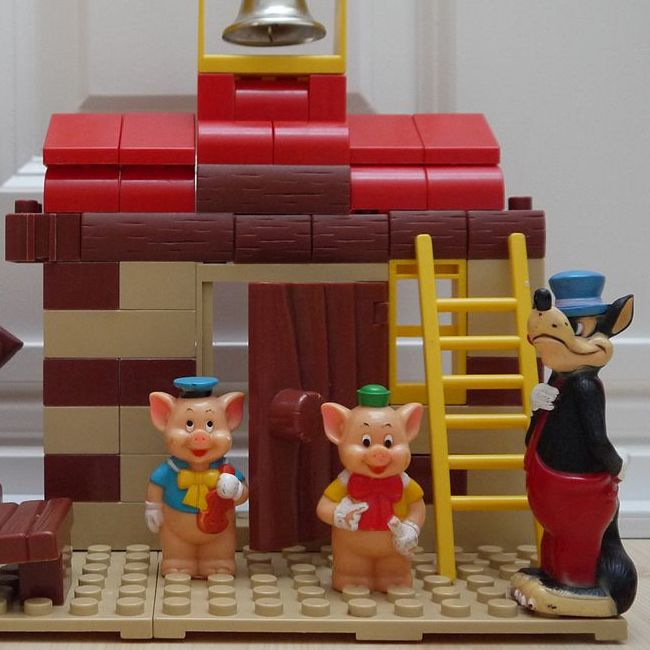The four simple ways to encode sum-types2019.11.06

There are four simple ways to encode sum types:

• Directly, if your programming language supports them
• "Church encoding"
• "Final style"
• The OO pattern

We'll introduce them and discuss their pros and cons, focusing on open (extensible) sum-types.

## Direct

All the up and coming programming languages support sum types, by feature if not by name:

Language Feature
F# Discriminated Unions
Elm Variants
Rust Enumerations
Swift Enumerations
Kotlin Sealed Classes

We'll use Haskell to demonstrate them:

``````data Shape
= Rect { width :: Float, height :: Float }
| Circle { radius :: Float }

area :: Shape -> Float
area (Rect w h) = w * h
area (Circle r) = pi * r * r

> area (Rect 3 5)
15``````

## Church encoding

How would we encode our type in legacy languages which don't support sum types?

Famous minimalist Alonzo Church has devised a method:

``````{-# LANGUAGE RankNTypes #-}

data ShapeHandlers r = ShapeHandlers
{ handleRect :: Float -> Float -> r
, handleCircle :: Float -> r
}

type Shape = (forall a. ShapeHandlers a -> a)

rect :: Float -> Float -> Shape
rect w h handlers = handleRect handlers w h

circle :: Float -> Shape
circle r handlers = handleCircle handlers r

area :: Shape -> Float
area shape =
shape
ShapeHandlers
{ handleRect = \w h -> w * h
, handleCircle = \r -> pi * r * r
}

> area (rect 3 5)
15``````

While originally intended for use in his minimal programming language "Lambda Calculus", this encoding is suitable for most popular languages. Java/C# supports it via abstract generic methods. In C++ or Go we'll have to resort to casts or side-effects to encode this (the Visitor pattern).

## Final style: Extending church-encodings using type classes

We can encode the record from the church encoding using a type-class:

``````{-# LANGUAGE RankNTypes #-}

class ShapeHandlers r where
rect :: Float -> Float -> r
circle :: Float -> r

type Shape = (forall a. ShapeHandlers a => a)

newtype Area = Area { area :: Float }

instance ShapeHandlers Area where
rect w h = Area (w * h)
circle r = Area (pi * r * r)

> area (rect 3 5)
15``````

The main benefit of using this encoding is that type class constraints are trivially composable, which translates to encoding extensible sum-types! For example: `(forall a. (ShapeHandlers a, MoreHandlers a) => a)`

With a small modification (avoiding universal quantification) this becomes Carette et al's "Final Style", which is also commonly known as "`mtl` style".

A similar encoding in OO languages, using interfaces instead of type classes is Oliviera et al's "Object Algebras".

## The OO pattern

This is a common way to represent sum types in object oriented languages:

``````data Rect = Rect { width :: Float, height :: Float }

data Circle = Circle { radius :: Float }

class Area a where
area :: a -> Float

instance Area Rect where
area (Rect w h) = w * h

instance Area Circle where
area (Circle r) = pi * r * r

> area (Rect 3 5)
15``````

This encoding is naturally open! We can add shapes as we please, and in posh languages which support type-classes or traits we can also add more operations on them without modifying existing code.

## Putting these approaches to the test

Let's explore how these approaches fare against some simple challenges.

### Supporting new shapes

Suppose we wanted to write code that can support more types of shapes, without modifying our shape data definition (aka the Expression problem).

The direct sum-type can't be extended, nor can its church encoding. But the final and OO styles can.

Final style:

``````class CompositeHandler r where
composite :: r -> r -> r

instance CompositeHandler Area where
composite (Area x) (Area y) = Area (x + y)

> area (composite (rect 3 5) (circle 1))
18.141592``````

OO style:

``````data Composite a b = Composite { first :: a, second :: b }

instance (Area a, Area b) => Area (Composite a b) where
area (Composite x y) = area x + area y

> area (Composite (Rect 3 5) (Circle 1))
18.141592``````

### Collections

If we wanted to encode a list of shapes:

• A final style list, `[(forall a. (ShapeHandlers a, CompositeHandler a) => a)]`, uses a universal quantifier and closes the list of supported variants
• An OO style list will have to use an existensial quantifier and close the list of supported operations

### Operations on more than one value

The `area` operation discussed earlier converts a given value to a result. But what if we wanted an operation that processes two shapes, like generating a diff of them?

This is where all styles discussed above fall short as far as I'm aware.

## The fifth approach: Composition of atoms

All the approaches discussed above failed when put to the test. The following approach fares better -

We build upon the OO approach's basic shapes and combine them into a concrete `Shape` sum type:

``````{-# LANGUAGE DeriveGeneric, DeriveAnyClass #-}

data Shape
= SRect Rect
| SCircle Circle
deriving (Generic, Area)``````

We use `Generic` and `DefaultSignatures` based derivations to derive our class instances (the derivation of `Area` is left as an exercise for the reader).

This approach allows us to implement our basic types, operations, and instances in a modular way, while only closing the type at the top-level. It does allow us to implement operations on more than one value (such as diffs), and we can encode a list in either of the styles supported by Final or OO styles.

Discussion:

•r/haskell

(image credit: MissMarvel50sWorld)